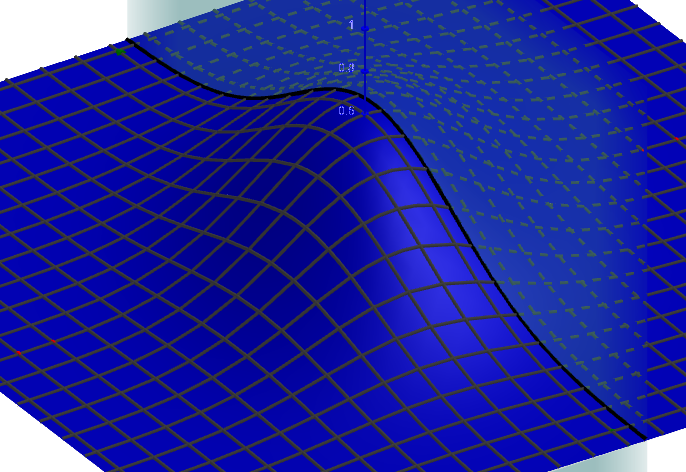# How can I plot a bivariate normal distribution in 3D to slice it to show conditional Expectation?

antoni.parellada shared this question 2 years ago1

```φ(x, μ, σ) = 1 / sqrt(2π σ) ℯ^((-(x - μ)²) / (2σ²))
a(x, y) = φ(x, 0, 1) φ(y, 0, 1)```

?1

Thank you, but these would be independent Gaussians, I believe. The idea is to have some correlation between them.1

then you have to give an example of what you are implying with "bivariate normal distribution"1

I found the equations I needed to generate it with Geogebra, I think, and if I can only bring back up the CAS column to finish typing them in, I feel optimistic that I'll be able to share. However, there is I don't understand what you mean by 'implication' in "bivariate normal distribution - a quick search returns on MathWorld, for example, exactly what I am saying: https://mathworld.wolfram.c...1

nothing wrong with that, the moment μ_{1}=μ_{2}=0 and σ_{1}=σ_{2}=1 , ρ=0 so it is the same

for other cases you have to calculate ρ, if you know how to do that easily let me know i want to learn too

```φ(x, μ, σ) = 1 / sqrt(2π σ) ℯ^((-(x - μ)²) / (2σ²))
φ_{xy}(x, y, μ_{1}, μ_{2}, σ_{1}, σ_{2}, ρ) = 1 / (2π σ_{1} σ_{1} sqrt(1 - ρ²)) ℯ^((-((x - μ_{1})² / σ_{1}² - (2ρ (x - μ_{1}) (y - μ_{2})) / (σ_{1} σ_{2}) + (y - μ_{2})² / σ_{2}²)) / (2 (1 - ρ²)))
f(x, y) = φ(x, 0, 1) φ(y, 0, 1)
g(x, y) = φ_{xy}(x, y, 0, 0, 1, 1, 0)```1

Exactly. Here is my approximation, but I wish I could make the z values more apparent visually with a gradient of colors.

https://www.geogebra.org/m/zrajyym72

Try with

```IntersectPath(b,p)
```

and line thickness higher1

I was actually trying exactly that - I am sure I learned it from you in the past. Thank you! Any way to do a color gradient for z values?2

unfortunately GGb does not support shading of lines or surfaces (coloured gradients or value dependent colorization) (although it would be a very cool feature)

the only option to achieve a similar effect is using trace paths with coded colour changes